CATEGORIES:

# Integration of simple rational fractions. Integration of rational fractions

LECTURE PLAN:

1. Selecting the proper rational fraction

2. Integrating Proper Rational Functions

3. Integrating Improper Rational Functions

Selecting the proper rational fraction

Suppose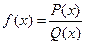is a rational function; that is,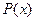andare polynomial functions. If the degree ofis greater than or equal to the degree of, then by long division,whereis a proper rational fraction; that is, the degree ofis less than the degree of. A theorem in advanced algebra states that every proper rational function can be expressed as a sum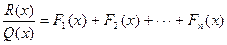whereare rational functions of the form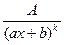or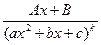in which the denominators are factors of. The sum is called the partial fraction decomposition of. The first step is finding the form of the partial fraction decomposition ofis to factorcompletely into linear and irreducible quadratic factors, and then collect all repeated factors so thatis expressed as a product of distinct factors of the form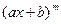and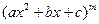.

From these factors we can determine the form of the partial fraction decomposition using the following two rules:

Linear Factor Rule: For each factor of the form, the partial fraction decomposition contains the following sum of m partial fractions: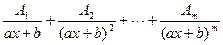where A1, A2, . . ., Am are constants to be determined.

Quadratic Factor Rule: For each factor of the form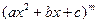, the partial fraction decomposition contains the following sum of m partial fractions: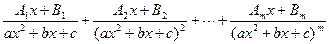where A1, A2, . . ., Am, B1, B2, …, Bm are constants to be determined.

I. Integrating Proper Rational Functions

Example 10: Find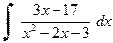.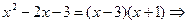using the Linear Factor Rule, we get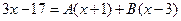after

multiplying by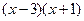. If we let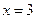, then; if we let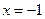, then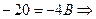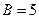. Thus,=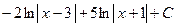.

Example 11: Find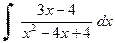.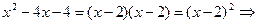by the Linear Factor Rule, we get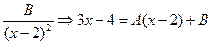after multiplying

by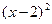. If we let x = 2, then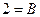; if we let x = 3, then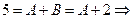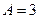. Thus,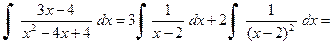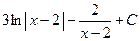.

II. Integrating Improper Rational Functions

Although the method of partial fractions only applies to proper rational functions, an improper rational function can be integrated by performing long division (or synthetic division). Ifis a rational function whereandare polynomial functions and the degree ofis greater than or equal to the degree of, then by long division,whereis a proper rational fraction. Sinceis a proper rational function, it can be decomposed into partial fractions.

Example 12: Find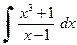.By synthetic division, 1 1 0 0 1.

1 1 11 1 1 2Thus,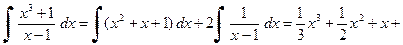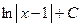.

Date: 2015-01-02; view: 1112

 <== previous page | next page ==> MAIN METHODS OF INTEGRATION | Type 5.
doclecture.net - lectures - 2014-2023 year. Copyright infringement or personal data (0.015 sec.)